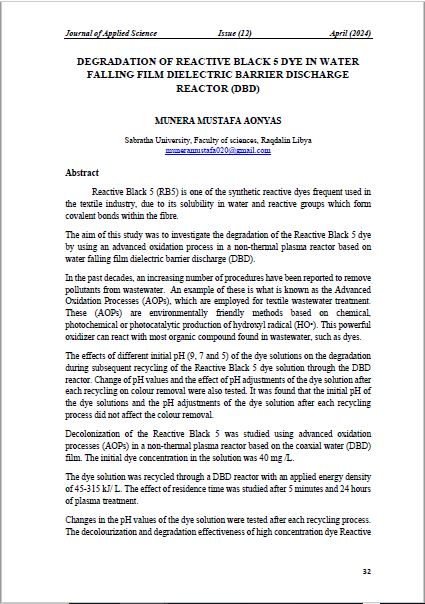# تأثير الشكل البياني للدالة على نتائج التكامل العددي المحدود للدوال الرياضية

• د. هناء المنير أحمد لوكه كلية التربية أبو عيسى / جامعة الزاوية
• أ. منى السنوسي محمد أبو بكر كلية التربية أبو عيسى / جامعة الزاوية

### Abstract

This paper view shed light on the graph of the function and its effect on results of limited numerical integration. Where it was taken four limited integrals to know the effect the graph of the function on the results of the limited integration، the first example is exponential function، the second example and third example are periodic triangle functions.

These functions are drawn by using Graph program، and we calculated these integrals using the direct mathematical method. Then we solved theses integrals using three numerical methods:  Trapezoidal rule، Simpson's rule، Romberg rule.

We calculated the absolute errors for these examples in order to make a comparison between the results using direct and numerical methods the results were rounded to four decimal places after the comma.

The results were written in tabular format to facilitate comparison، to compute the numerical integration of the periodic functions we found the Romberg method gives very accurate results if all the values (x، y) located in the first quarter the results of the numerical integral are very accurate and their convergence with the real values of the integration is the best، after that comes Trapezoidal method.

### Author Biographies

د. هناء المنير أحمد لوكه, كلية التربية أبو عيسى / جامعة الزاوية

كلية التربية أبو عيسى / جامعة الزاوية

أ. منى السنوسي محمد أبو بكر, كلية التربية أبو عيسى / جامعة الزاوية

كلية التربية أبو عيسى / جامعة الزاويةPublished
2020-09-27
How to Cite
لوكهد. ه., & أبو بكرأ. م. (2020). تأثير الشكل البياني للدالة على نتائج التكامل العددي المحدود للدوال الرياضية. Scientific Journal of Applied Sciences of Sabratha University, (issue 5), 118-138. Retrieved from https://jas.sabu.edu.ly/index.php/asjsu/article/view/114
Section
Articles